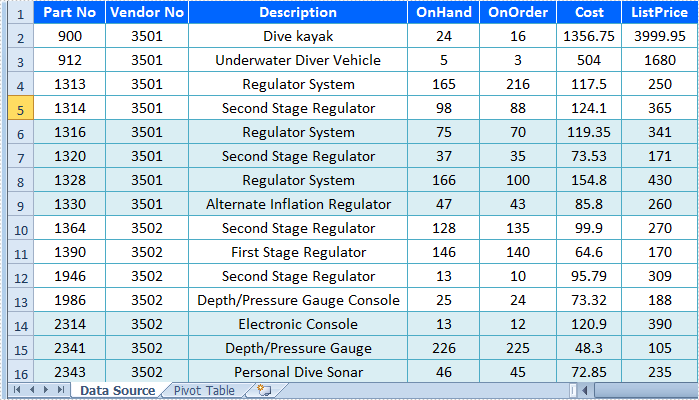# Create Pivot Table in Excel in C#, VB.NET

Pivot table is one kind of interactive table, which can be used to calculate data, such as get sum or count data. Also, users can change pivot table layout for analyzing data with different ways or reassign row/column label. Every time users change layout, data will be recalculated in pivot table. Also, pivot table can be updated if the source data is changed.

This program guide focuses on introducing how to create Pivot Table in Excel by using C#/VB.NET via Spire.XLS.

In this guide, there is an Excel file which includes a worksheet with data information. What we will do is to add a new worksheet and create pivot table in this worksheet. Data source of pivot table is from the original worksheet.

Step 1: Load Excel File

Declare a new Excel workbook and then load file by using workbook.LoadFromFile() method. Then, initialize worksheet and name the current worksheet. Because we need to create pivot table in another worksheet, so create a new worksheet and name it.

[C#]
```Workbook workbook = new Workbook();
workbook.LoadFromFile(@"E:\work\Documents\PartSalesInfo.xlsx");
Worksheet sheet = workbook.Worksheets;
sheet.Name = "Data Source";
Worksheet sheet2 = workbook.CreateEmptySheet();
sheet2.Name = "Pivot Table";
```
[VB.NET]
```Dim workbook As New Workbook()
workbook.LoadFromFile("E:\work\Documents\PartSalesInfo.xlsx")
Dim sheet As Worksheet = workbook.Worksheets(0)
sheet.Name = "Data Source"
Dim sheet2 As Worksheet = workbook.CreateEmptySheet()
sheet2.Name = "Pivot Table"
```

Step 2: Create Pivot Table

At first, add pivot table. Select data source range which is from the original worksheet. Create a PivotCache to save the data information. Next, create a pivot table in the new worksheet. Assign value for pivot table by using sheet2.PivotTables.Add() method. Three parameters passed to this method, name string, table location and pivot cache.

[C#]
```CellRange dataRange = sheet.Range["A1:G17"];
PivotCache cache = workbook.PivotCaches.Add(dataRange);
PivotTable pt = sheet2.PivotTables.Add("Pivot Table", sheet.Range["A1"], cache);
```
[VB.NET]
```Dim dataRange As CellRange = sheet.Range("A1:G17")
Dim cache As PivotCache = workbook.PivotCaches.Add(dataRange)
Dim pt As PivotTable = sheet2.PivotTables.Add("Pivot Table", sheet.Range("A1"), cache)
```

Secondly, define row labels. We can set some data information as row label to assign data information. Get PivotField of the first row label we will set and then set its AxisType as row. After that, set header name. Next, set the second row label as setting the first one but without header name.

[C#]
```var r1 = pt.PivotFields["Vendor No"];
r1.Axis = AxisTypes.Row;
pt.Options.RowHeaderCaption = "Vendor No";

var r2 = pt.PivotFields["Description"];
r2.Axis = AxisTypes.Row;
```
[VB.NET]
```Dim r1 = pt.PivotFields("Vendor No")
r1.Axis = AxisTypes.Row
pt.Options.RowHeaderCaption = "Vendor No"

Dim r2 = pt.PivotFields("Description")
r2.Axis = AxisTypes.Row
```

Thirdly, add new data fields and set format. Besides the original data information, we can add more fields to calculate data by using pt.DataFileds.Add() method. There are three parameters passed to this method, Pivot Field, name string and subtotal type. The fields we will add include SUM of OnHand, SUM of OnOrder and Average of ListPrice. Finally, set built-in style for table.

[C#]
```pt.DataFields.Add(pt.PivotFields["OnHand"], "SUM of OnHand", SubtotalTypes.Sum);
pt.DataFields.Add(pt.PivotFields["OnOrder"], "SUM of OnOrder", SubtotalTypes.Sum);
pt.DataFields.Add(pt.PivotFields["ListPrice"], "Average of ListPrice", SubtotalTypes.Average);

pt.BuiltInStyle = PivotBuiltInStyles.PivotStyleMedium12;
```
[VB.NET]
```pt.DataFields.Add(pt.PivotFields("OnHand"), "SUM of OnHand", SubtotalTypes.Sum)
pt.DataFields.Add(pt.PivotFields("OnOrder"), "SUM of OnOrder", SubtotalTypes.Sum)
pt.DataFields.Add(pt.PivotFields("ListPrice"), "Average of ListPrice", SubtotalTypes.Average)

pt.BuiltInStyle = PivotBuiltInStyles.PivotStyleMedium12
```

Step 3: Save and Launch

Save this Excel file which has been added pivot table by using workbook.SaveToFile() method. There are two parameters passed to this method, file name string and Excel version. In this example, Excel version is set as Version2010. Then, launch it for viewing.

[C#]
```workbook.SaveToFile("PivotTable.xlsx", ExcelVersion.Version2010);
System.Diagnostics.Process.Start("PivotTable.xlsx");
```
[VB.NET]
```workbook.SaveToFile("PivotTable.xlsx", ExcelVersion.Version2010)
System.Diagnostics.Process.Start("PivotTable.xlsx")
```

Effective Screeshot

Data Source:Pivot Table: